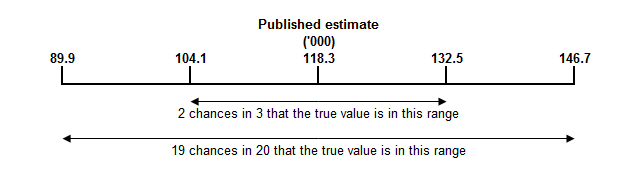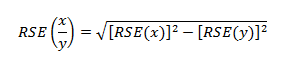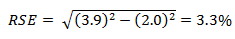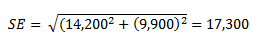6238.0 - Retirement and Retirement Intentions, Australia, July 2016 to June 2017
ARCHIVED ISSUE Released at 11:30 AM (CANBERRA TIME) 18/12/2017
 Page tools: .mffeedback,#pjs { display :none; } Enable Javascript to Print PagesPrint All TECHNICAL NOTE DATA QUALITY INTRODUCTION 1 Since the estimates published in this publication are based on information obtained from occupants of a sample of households, they are subject to sampling variability. That is, they may differ from those estimates that would have been produced if all households had been included in the survey. One measure of the likely difference is given by the standard error (SE), which indicates the extent to which an estimate might have varied by chance because only a sample of households (or occupants) was included. 2 There are about two chances in three (67%) that a sample estimate will differ by less than one SE from the number that would have been obtained if all households had been included, and about 19 chances in 20 (95%) that the difference will be less than two SEs. 3 Another measure of the likely difference is the relative standard error (RSE), which is obtained by expressing the SE as a percentage of the estimate. RSE% = (SE/estimate) x 100 4 RSEs for Retirement and Retirement Intentions estimates have been calculated using the Jackknife method of variance estimation. This process involves the calculation of 30 'replicate' estimates based on 30 different subsamples of the original sample. The variability of estimates obtained from these subsamples is used to estimate the sample variability surrounding the main estimate. 5 The Excel spreadsheets in the Downloads tab contain all the tables produced for this release and the calculated RSEs for each of the estimates. The RSEs for estimates other than medians have been calculated using the Jackknife method, and RSEs for the medians have been calculated using the Woodruff method. 6 In the tables in this publication, only estimates (numbers, percentages, means and medians) with RSEs less than 25% are considered sufficiently reliable for most purposes. However, estimates with larger RSEs have been included. Estimates with an RSE in the range 25% to 50% should be used with caution while estimates with RSEs greater than 50% are considered too unreliable for general use. All cells in the Excel spreadsheets with RSEs greater than 25% contain a comment indicating the size of the RSE. These cells can be identified by a red indicator in the corner of the cell. The comment appears when the mouse pointer hovers over the cell. CALCULATION OF STANDARD ERROR AND RELATIVE STANDARD ERROR 7 RSEs are routinely presented as the measure of sampling error in this publication and related products. SEs can be calculated using the estimates (counts or means) and the corresponding RSEs. 8 An example of the calculation of the SE from an RSE follows. Datacube 3 shows that the estimated number of females aged 55–59 who retired from the labour force aged less than 55 years is 118,300 and the RSE for this estimate is 12.0%. The SE is: SE of estimate = (RSE / 100) x estimate = 0.12 x 118,300 = 14,200 (rounded to the nearest 100) 9 Therefore, there are about two chances in three that the value that would have been produced if all households had been included in the survey will fall within the range 104,100 to 132,500 and about 19 chances in 20 that the value will fall within the range 89,900 to 146,700. This example is illustrated in the following diagram.PROPORTIONS AND PERCENTAGES 1247 10 Proportions and percentages formed from the ratio of two estimates are also subject to sampling errors. The size of the error depends on the accuracy of both the numerator and the denominator. A formula to approximate the RSEs of proportions not provided in the spreadsheets is given below. This formula is only valid when x is a subset of y.11 Considering Datacube 3, of the 1,943,800 females who were retired from labour force, 898,300 or 46.2% were aged less than 55 years at retirement. The RSE of 898,300 is 3.9% and the RSE for 1,943,800 is 2.0%. Applying the above formula, the RSE for the proportion of females who retired aged less than 55 years is:12 Therefore, the SE for the proportion of females who retired from the labour force aged less than 55 years is 1.5 percentage points (= (46.2/100) x 3.3). Therefore, there are about two chances in three that the proportion of females who retired from the labour force aged less than 55 years is between 44.7% and 47.7%, and 19 chances in 20 that the proportion is within the range 43.2% to 49.2%. SUMS OR DIFFERENCES BETWEEN ESTIMATES 13 Published estimates may also be used to calculate the sum of, or difference between, two survey estimates (of numbers, means or percentages) where these are not provided in the spreadsheets. Such estimates are also subject to sampling error. 14 The sampling error of the difference between two estimates depends on their SEs and the relationship (correlation) between them. An approximate SE of the difference between two estimates (x–y) may be calculated by the following formula:15 The sampling error of the sum of two estimates is calculated in a similar way. An approximate SE of the sum of two estimates (x+y) may be calculated by the following formula:16 An example follows. From paragraph 8 the estimated number of females aged 55–59 who retired from the labour force aged less than 55 years is 118,300 and the SE is 14,200. From Datacube 3, the estimate of females aged 60–64 who retired from the labour force aged less than 55 years is 124,700, the RSE is 7.9% and the SE is 9,900 (rounded to nearest 100). The estimate of females aged 55–64 who retired from the labour force aged less than 55 years is: 118,300 + 124,700 = 243,000 17 The SE of the estimate of females aged 55–64 who retired from the labour force aged less than 55 years is:18 Therefore, there are about two chances in three that the value that would have been produced if all households had been included in the survey will fall within the range 225,700 to 260,300 and about 19 chances in 20 that the value will fall within the range 208,400 to 277,600. 19 While these formulae will only be exact for sums of, or differences between, separate and uncorrelated characteristics or subpopulations, it is expected to provide a good approximation for all sums or differences likely to be of interest in this publication. SIGNIFICANCE TESTING 20 A statistical test for any comparisons between estimates can be performed to determine whether it is likely that there is a significant difference between two corresponding population characteristics. The standard error of the difference between two corresponding estimates (x and y) can be calculated using the formula in paragraph 10. This standard error is then used to calculate the following test statistic:21 If the value of this test statistic is greater than 1.96 then there is evidence, with a 95% level of confidence, of a statistically significant difference in the two populations with respect to that characteristic. Otherwise, it cannot be stated with confidence that there is a difference between the populations with respect to that characteristic. 22 The imprecision due sampling variability, which is measured by the SE, should not be confused with inaccuracies that may occur because of imperfections in reporting by respondents and recording by interviewers, and errors made in coding and processing data. Inaccuracies of this kind are referred to as non-sampling error, and they occur in any enumeration, whether it be a full count or sample. Every effort is made to reduce non-sampling error to a minimum by careful design of questionnaires, intensive training and supervision of interviewers, and efficient operating procedures. Document Selection These documents will be presented in a new window.